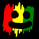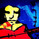1421 뷰
1421
is the Hull Moving Average Cross (simple for Alert friendliness)
```//@version=2
//                            is the Hull Moving Average Cross (simple for Alert friendliness) by SeaSide420
study("HMA_X", overlay=true)
jah=input(title="HullMA cross",type=integer,defval=14)
n2ma=2*wma(close,round(jah/2))
nma=wma(close,jah)
diff=n2ma-nma
sqn=round(sqrt(jah))
n2ma1=2*wma(close,round(jah/2))
nma1=wma(close,jah)
diff1=n2ma1-nma1
sqn1=round(sqrt(jah))
n1=wma(diff,sqn)
n2=wma(diff1,sqn)
cannabist  =n1>n2?green:black
of         =n1>n2?black:red
cantheist  =n1>n2?green:red
n1e=plot(n1, title="HMA1", color=cannabist, linewidth=2, offset=2)
n2e=plot(n2, title="HMA2", color=of, linewidth=2, offset=2)
fill(n1e, n2e, color=cantheist, transp=80)// o_O // hi! :-)```marklane
@marklane,
if yu right click on TV chart
응답seaside being most toxic coder on this site; nice to see you still being aids
응답MarxBabu
mate.....